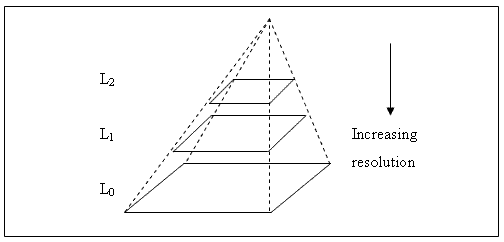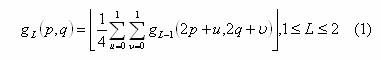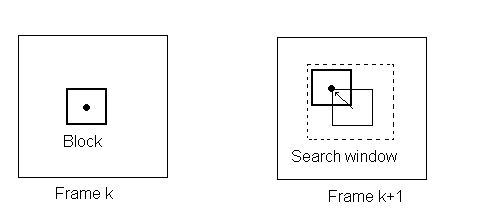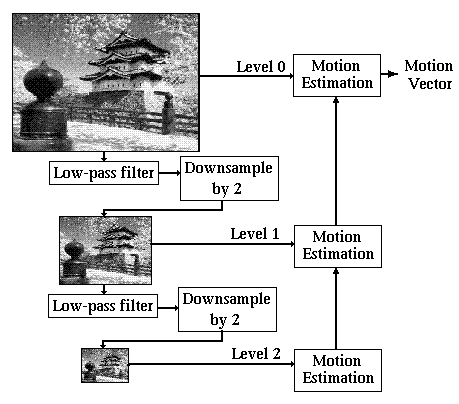Hierarchical Estimation of the motion vector field

Hierarchical estimation of the motion vector field (also known as or pyramid search) is a widely applied approach to motion estimation. It offers low computational complexity and high efficiency, plus a large degree of flexibility in the trade-off between the two.

Other approaches to motion field estimation include the full search algorithm and the various heuristic algorithms. In full search, each block of pixels in one frame is compared to every possible block in the next frame to find the best match, and the corresponding motion vector.

In heuristic algorithms, only a selected number of blocks are compared to the initial block, based on a certain assumption on the form of the vector field. Such algorithms include the three-step search (), four-step search (), and the Diamond Search (, ).

The full-search algorithm offers high quality results at a forbidding computational cost, while the results from the heuristic algorithms are often poor. The hierarchical algorithm is a newer, both fast and efficient approach.

In hierarchical estimation, both frames undergo a process of size and resulotion reduction. Several levels are constructed, each containing the same image as the previous level, having both dimensions reduced by a certain factor (usually 2). The result is a pyramid, where the lowest level is the initial image, and each level above it is the same image at ¼ of its size. (Fig. 1).Fig. 1: The hierarchical (pyramid) structure of an image.

In order to create a lower resolution image from the initial one, two approaches can be used: The mean intensity (), or subsampling.

In the case of grey-level images, for the mean intensity approach, each block of (usually) four pixels is replaced by one, having their mean intensity. That is:where gL(p,q) is the pixel intensity of pixel (p,q) of the Lth level, and g0(p,q) denotes pixel (p,q) of the original image.

Subsampling is another popular approach for reducing an image’s size. During subsampling, each block of pixels is replaced by only one of them (e.g. the upper leftmost). This approach yields lower quality results than the mean intensity method, but is significantly faster.

After the pyramid has been created for both images, the corresponding higher-level block is located, for each 0-level block of the first frame. A full search then takes place in the higher level of the second frame. This means that a search window is defined in the second frame, and, for each block in the first frame all candidate motion vectors are evaluated. This is achieved by comparing all the blocks in the search window to the block in the first frame whose vector is sought (Fig. 2).Fig. 2: Motion vector estimation with the full-search algorithm

In order to compare blocks, a measure of the block difference has to be established. The most widely used block distance measure is the Mean Absolute Difference:where (m, n) are the block dimensions, gf(k, l) signifies the intensity of pixel (k, l) in frame f, and (i, j) is the candidate motion vector. Other Block Distance Measures include the Mean Square Difference, and the Pixel Difference Classification .

It was mentioned above that the full-search algorithm is computationally expensive. This does not apply to our case, however, since the resolution reduction gives us the ability to search a large area of the image for the best match, without having to perform too many comparisons.

The full-search algorithm on the highest level gives a crude estimation of the motion vector. In order to get the final vector, the initial estimation will have to be refined for each consecutive level. There are various approaches in order to achieve this.

A very common method is to propagate the result to the next level, and perform a heuristic search in a small area to locate the best match for this level. , [8, pg 106]. The vector is propagated to the next level by multiplying both its coordinates with the scaling factor (in most cases, 2). The process of propagating the vector and refining it is repeated until a result for level 0 is reached, where the process ends.

Another approach to the refinement of the motion vector is to take into account only the 4 level L-1 blocks that result from 1 level L block (). This approach is definitely faster, but also less reliable.

It is also possible to perform a full search anew at each level, in a small search window around the initial estimation ().

A common problem that arises in hierarchical search is that an erroneous match in the higher level is almost always propagated to the lower levels, and is bound to result in an erroneous motion vector. This is not a rare case at all, since the low resolution of the highest level often leads to ambiguity. That is, a number of candidate blocks will have similar Block Distance Measure values, and the one being actually matched will be slightly better than the others in the higher level, but not necessarily better at level 0. To prevent this, it is possible to propagate more than one vector down to level 1 (, ). If a number of blocks with similar BDMs appear at the highest level, all of them can be refined for every lower level and the best one selected among them. The increase in computational cost is small compared to the increase in the quality of the results.

In all, the hierarchical motion field estimation offers high quality results, at a very low computational cost, and the large number of parameters to be specified, (block size, number of levels, scaling factor) as well as its many variations make it one of the most widely used algorithm for a wide range of applications besides motion estimation, including video coding   and stereo vision .Fig. 3: Flowchart of the generic Hierarchical Motion Field Estimation algorithm